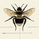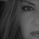39534 뷰
This is my custom channel (Bear Channel!? :)) derived from BB/ STARC . It uses both ATR/STDEV for plotting the bounds.

I use COG (Center of Gravity ) for deriving the baseline. This enables it to track the price action better than many other channels that make use of MAs or simply "close". Indicator also marks "squeezes" (stdev bands come inside ATR bands). Pay attention to these, as these usually indicate a move.

I am still exploring this indicator on different BTCUSD time frames, would love to hear your feedback / setups for other instruments.

Code for this indicator: http://pastebin.com/QXBJqAhA

Code for COG fibs I mentioned in the comments: http://pastebin.com/CbxY31at
오픈 소스 스크립트

진정한 TradingView의 정신에 따라, 이 스크립트의 작성자는 스크립트를 오픈소스로 게시했기에 거래자들이 이해하고 확인할 수 있습니다. 작가님께 건배! 스크립트를 무료로 사용할 수 있지만, 게시물에서 이 코드를 재사용하는 것은 하우스룰을 따릅니다. 당신은 스크립트를 차트에 사용하기 위해 그것을 즐겨찾기 할 수 있습니다.

차트에 이 스크립트를 사용하시겠습니까?
```//
// @author LazyBear
//
// This is my custom channel derived from BB/STARC. This uses both ATR/STDEV for plotting the bounds.
// I use COG for the base line (normally it is SMA/EMA).
//
// If you use this code in its original/modified form, do drop me a note.
//
study("COG Double Channel [LazyBear]", shorttitle="COGChannel_LB", overlay=true)
src = close
length = input(34)
median=0
mult=input(2.5)
offset = input(20)
tr_custom() =>
x1=high-low
x2=abs(high-close)
x3=abs(low-close)
max(x1, max(x2,x3))

atr_custom(x,y) =>
sma(x,y)

dev = (mult * stdev(src, length))
basis=linreg(src, length, median)
ul = (basis + dev)
ll = (basis - dev)
tr_v = tr_custom()
acustom=(2*atr_custom(tr_v, length))
uls=basis+acustom
lls=basis-acustom

// Plot STDEV channel
plot(basis, linewidth=1, color=navy, style=line, linewidth=1, title="Median")
lb=plot(ul, color=red, linewidth=1, title="BB+", style=dashed)
tb=plot(ll, color=green, linewidth=1, title="BB-", style=dashed)
fill(tb,lb, silver, title="Region fill")

// Plot ATR channel
plot(basis, linewidth=2, color=navy, style=line, linewidth=2, title="Median")
ls=plot(uls, color=red, linewidth=1, title="Starc+", style=circles)
ts=plot(lls, color=green, linewidth=1, title="Star-", style=circles)
fill(ts,tb, green, title="Region fill")
fill(ls,lb, red, title="Region fill")

// Mark SQZ
plot_offs_high=2
plot_offs_low=2
sqz_f=(uls>ul) and (lls<ll)
b_color=sqz_f ? teal : na
plot(sqz_f ? lls-plot_offs_low : na, color=b_color, style=cross, linewidth=2)
plot(sqz_f ? uls+plot_offs_high : na, color=b_color, style=cross, linewidth=2)
```
List of my free indicators: http://bit.ly/1LQaPK8
List of my indicators at Appstore: http://blog.tradingview.com/?p=970

## 코멘트Kurbelklaus
응답Something like this works better for all symbols, rather than a hard set 2:

plot_offs_high=0.002
plot_offs_low=0.002
sqz_f=(uls>ul) and (lls<ll)
b_color=sqz_f ? teal : na
plot(sqz_f ? lls - (lls * plot_offs_low) : na, color=b_color, style=cross, linewidth=2)
plot(sqz_f ? uls + (uls * plot_offs_high) : na, color=b_color, style=cross, linewidth=2)
응답LazyBear
NP, thanks for this and all the rest
응답LazyBear
Can you update this tool with TheLark correction? I have no idea of how to do it. Thank you
응답sublimares2
Pastebin link I have given above has this.
응답LazyBear
If only we had a screener to find these Sqz plays.
응답Quick visual comparison of this channel with BB. Similar ATR comparison can be made too.

응답